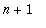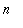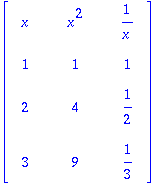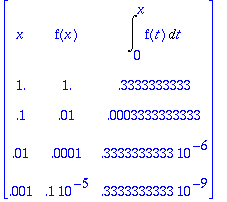Function: chart - show a table of function values

Calling Sequences:

Parameters:

heading - a list of expressions, each depending on the first member of the list

values - a list of expressions, which are to be substituted for the first member of heading .

flag - a boolean value.

Description:

This prints a table of values of one or more expressions (using "table" in the ordinary sense of the word, rather than its technical meaning in Maple), in the form of a matrix.

The first row of the matrix is the value of heading . This is a list of expressions, each of which contains the first entry (which is typically a variable x ).

Rowof the matrix, forfrom 1 to the number of items in values , is obtained by substituting values[n] for x in heading and evaluating it.

If the third argument flag is omitted or true, then each entry is evaluated numerically using evalf .

If a member of heading is a function that should only be evaluated with numerical arguments, you can delay evaluation by placing single quotes around it, e.g. 'f(x)' .

This function is part of the Maple Advisor Database library, and must be loaded before use by the command readlib(chart); .

Examples:

> chart([x, x^2, 1/x],[1,2,3],false);> chart([x, x^2, 1/x], [1,2,3]);> f:= x -> x^2:

> chart([x, 'f(x)', Int('f(t)', t = 0 .. x)],[1, .1, .01, .001]);